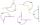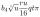# Cube 5

The surface of the cube is 15.36 dm2. How will change the surface area of this cube if the length of the edges is reduced by 2 cm?

Result

S =  11.76 dm2
S1 - S2 =  3.6 dm2

#### Solution:Leave us a comment of example and its solution (i.e. if it is still somewhat unclear...):Be the first to comment!## Next similar examples:

1. Cube 2How many times will increase if the surface area of the cube if we triple length of its edge?
2. CubeHow many times increases the surface area of a cube with edge 23.4 cm if the length of the edge doubled?
3. Cube edgesThe sum of the lengths of the cube edges is 42 cm. Calculate the surface of the cube.
4. CubeThe sum of all cube edges is 30cm. Find the surface area of the cube.
5. Cube edgeDetermine the edges of the cube when the surface is equal to 37.5 cm square.
6. Cube surface areaWall of cube has content area 99 cm square. What is the surface of the cube?
7. Simplify 2Simplify expression: 5ab-7+3ba-9
8. ExpressionSolve for a specified variable: P=a+4b+3c, for a
9. AlleyAlley measured a meters. At the beginning and end are planted poplar. How many we must plant poplars to get the distance between the poplars 15 meters?
10. SimplifySimplify the following problem and express as a decimal: 5.68-[5-(2.69+5.65-3.89) /0.5]
11. HotelThe hotel has a p floors each floor has i rooms from which the third are single and the others are double. Represents the number of beds in hotel.
12. Complementary anglesTwo angles are complementary. If one angle measures 10°, what is the measure of the second angle?
13. ExpressionsExpression (3a-7).(3a+7) is identical with: ?
14. 8 degrees8 degrees Celsius it cools down by 9 degrees Celsius. What is the resultant temperature?
15. HostelStudents are accommodated in 22 rooms. Rooms were 4 and 6 bed. How many rooms in which type occupied 106 children there?
16. Greg and BillGreg is 18 years old. He is 6 less than 4 times Bill's age. How old is Bill?
17. One-thirdA one-third of unknown number is equal to five times as great as the difference of the same unknown number and number 28. Determine the unknown number.